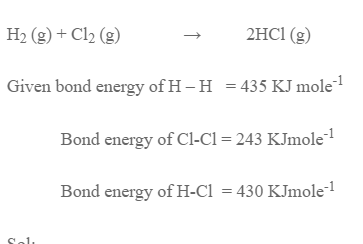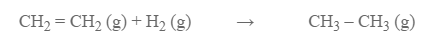# Bond Energy Or Bond Enthalpy

Bond energy of a bond is amount of energy required to break 1 mole of a bond in gaseous state or energy released when one mole of a bond is formed in gaseous state.

In terms of bond energy,
Hreaction = Heat absorbed – Heat released = bond energies of reactants – bond energies of products

Estimate the enthalpy change for the reactionSol:
The amount of energy released during formation of 2moles of H-Cl bond = 2 × 430 = 860 KJ

H reaction = Energy absorbed – Energy released
= 618 – 860
= -182 KJ

Calculate the enthalpy change for the hydrogenation of ethane gas.Given, bond energy of H -H bond = 435 KJ mole-1

Bond energy of C – H bond = 413 KJ mole-1

Bond energy of C -C bond = 347 KJ mole-1

Bond energy of C=C bond = 615 KJ mole-1

The amount of energy absorbed during reaction
= 413 × 4 + 615 + 435
= 2702 KJ

Energy released during reaction
= 6 × 413 + 347
= 2825 KJ

H reaction = 2825 – 2702 KJ = -123 KJ